## The Isoperimetric Inequality

Given a rope of length L, say 100 meters, what is the largest closed area you can make?
If we try a square, each side has to be L/4 (25 meters), and we'll end up with an area of (L/4)^2 = 625 square meters. If we however try a circle, the circumference is L=2πR, where R is the radius. Thus R = L/(2π), and the area is π(L/2π)^2 = L^2/(4π) = 796 square meters, which is better than the square.

But there's plenty more shapes to try, in fact, infinitely many. So which should we pick to maximize the area?
The ancients knew the answer but could not prove it: it's the circle. The proof of this is surprisingly simple using calculus. Let L be the length of the rope, and let A be the enclosed area.

Note that for a circle, the relationship between A and L is L=2πR and A=πR^2, where R is the radius, so that: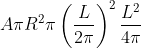Given the belief that the circle gives the largest area, we expect that for any shape,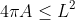This is known as the isoperimetric inequality.

## Hurwitz's Proof

Assume that the curve (x(t),y(t)) is parametrized so that it does one full revolution when t goes from 0 to π. The arc length is defined by s = Lt/(2π). Note that when t=0, s=0, and when t=2π, s=L.

We then have: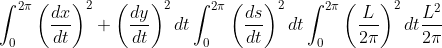As for the area, we use Green's theorem: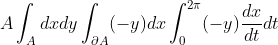Now, simply take the difference we want to show is always non-negative:We want to conclude that this expression is non-negative for any curve. The first integral is clearly non-negative, since its integrand is a square. As for the second integral, the result is not obvious until one consider its Fourier series (this is called Wirtinger's inequality):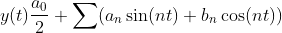As we will see soon, for this to work, we must place the curve in coordinates such that: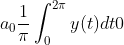This can always be done, as the placement of the curve is arbitrary: if the integral of y(t) is V, then the translated function y(t)-V/(2π) will have integral 0.

Next, differentiate y(t) to obtain: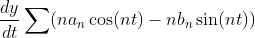Then, by Parseval's identity: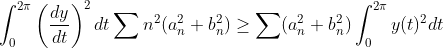which concludes the proof. (The requirement a0=0 is to ensure that the last inequality holds for n=0).
The inequality thus obtained is known as the isoperimetric inequality:## Only the Circle Yields Maximum Area

As we saw in the beginning, the circle turns the inequality into an equality, thus yielding the maximum possible area. But this still leaves open the possibility that other shapes may be equally good. We can show that only the circle yields max area by considering when the inequality becomes an equality: precisely when the two integrals are zero.

Starting with the second integral, the inequality was obtained from comparing the square sums after applying Parseval's identity. To have equality, the terms in those sums must be 0 except when n=1, which means that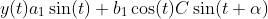The first integral must also be zero, which means that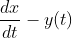Integrating, we get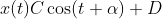So indeed we must have a circle centered at (D,0).

The fact that the circle must be centered around y=0 is due to our previous choice of fixing the integral of y(t) over one period. The center of mass must be such that equally much is positive and negative, which for a circle clearly means fixing it around y=0.

## How general is this proof?

The use of Green's theorem requires that we deal with a curve (x(t),y(t)) that has a continuous derivative except in finitely many points (piecewise smooth). Piecewise smoothness is also enough to ensure that the Fourier series can be differentiated term by term. Any area you can physically construct will certainly satisfy these conditions, as will anything you draw with a pen, since it is impossible to create or draw infinitely many edged points.

## Monday, October 21, 2013

### How to iterate over the values of a std::map

EDIT: Changed example to better reflect the generality of the problem = title of this post. END EDIT.

Now that C++ has lambdas, the C++ STL algorithms are practically useful, but how do you iterate over the values of a std::map?

Say you have a function template:

template <typename Iter> void f(Iter curr, Iter end);

f assumes the iterators point to std::strings:

std::vector<std::string> v;

f(v.begin(),v.end()); // ok


std::map<int,std::string> m;
f(m.begin(),m.end()); // Won't work: *m.begin() is a pair<Key,Value>

What we thus need is a way of converting the map::iterator (and map::const_iterator) to an iterator to always dereferences the Value type of the pair.

## Solution using Boost

The solution is easy using boost:

#define BOOST_RESULT_OF_USE_DECLTYPE
#include <boost/iterator/transform_iterator.hpp>

// First, define a lambda to get the second element of a pair:
auto get_second = [](const std::pair<const int,std::string>& p){ return p.second; };

// Then, we can convert a map iterator into an iterator that automatically dereferences the second element
auto beg = boost::make_transform_iterator(m.begin(),get_second);
auto end = boost::make_transform_iterator(m.end(),get_second);

f(beg,end); // ok, works!


The line
#define BOOST_RESULT_OF_USE_DECLTYPE
is needed so inform the boost libraries that the result type (Value in this case) should be inferred using decltype(), rather than by requiring a result_of typedef (prior to C++11, decltype did not exist).

## Solution without Boost

If you can't use boost, you'll need to implement the dereferencing by hand. Here's the code:

#include <map>
#include <iterator>

template <typename Iter>
class map_iterator : public std::iterator<std::bidirectional_iterator_tag,typename Iter::value_type::second_type> {
public:
map_iterator() {}
map_iterator(Iter j) : i(j) {}
map_iterator& operator++() { ++i; return *this; }
map_iterator operator++(int) { auto tmp = *this; ++(*this); return tmp; }
map_iterator& operator--() { --i; return *this; }
map_iterator operator--(int) { auto tmp = *this; --(*this); return tmp; }
bool operator==(map_iterator j) const { return i == j.i; }
bool operator!=(map_iterator j) const { return !(*this == j); }
reference operator*() { return i->second; }
pointer operator->() { return &i->second; }
protected:
Iter i;
};

template <typename Iter>
inline map_iterator<Iter> make_map_iterator(Iter j) { return map_iterator<Iter>(j); }

// We can now do:

 std::map<int,std::string> m;
 // ...
f(make_map_iterator(m.begin()),make_map_iterator(m.end()));






## STL Container Performance Table

There's already a good table at Stack Overflow listing time complexity (in Big O notation) of common operations with C++ STL containers for comparison, although it's structured in a more abstract way and a little hard to read because it's not a HTML table. Here's my version, which also includes priority_queue (technically an adaptor).

Persistent iterators means that the iterators are not invalidated by insertions and erase (except when erasing the element referred to by the iterator, which is necessarily invalidated).

ContainerInsertionAccessEraseFindPersistent Iterators
vector / stringBack: O(1) or O(n)
Other: O(n)
O(1)Back: O(1)
Other: O(n)
Sorted: O(log n)
Other: O(n)
No
dequeBack/Front: O(1)
Other: O(n)
O(1)Back/Front: O(1)
Other: O(n)
Sorted: O(log n)
Other: O(n)
Pointers only
list / forward_listBack/Front: O(1)
With iterator: O(1)
Index: O(n)
Back/Front: O(1)
With iterator: O(1)
Index: O(n)
Back/Front: O(1)
With iterator: O(1)
Index: O(n)
O(n)Yes
set / mapO(log n)-O(log n)O(log n)Yes
unordered_set / unordered_mapO(1) or O(n)O(1) or O(n)O(1) or O(n)O(1) or O(n)Pointers only
priority_queueO(log n)O(1)O(log n)--

## Always O(1): begin(), end(), empty(), size(), push_back()

The following operations are always O(1) when they exist:
1. begin(), end()
2. empty()
3. size() (note that list::size() was not necessarily O(1) prior to C++11)
4. push_front() (note that std::vector does not have push_front(), as it would not be O(1))
5. push_back()

For std::queue and std::stack, complexity depends on the underlying container used (by default std::deque).

### vector

std::vector has constant time (O(1)) back insertion provided no reallocation needs to take place (use reserve/capacity to allocate/check). When reallocation is necessary, all elements are copied (or moved, if possible) to a need memory location. It is guaranteed that back insertion is amortized constant, meaning: "if we perform a large amount of back insertions, the average time for back insertion is constant".

Insertion does not invalidate iterators as long as no reallocation is performed (when reallocating, all iterators become invalid). Deletion invalidates all iterators after the deleted element, iterators to elements before are still valid.

### deque

Insertion and deletion of elements in a std::deque may invalidate all its iterators. Pointers are however persistent. In practice accessing / iterating over a std::vector is faster than std::deque.

All iterators may become invalid after an insertion or deletion, but pointers/references are always valid.

### list

If you have an iterator to an element, you can insert right after (or before) that element in constant time (O(1)). Of course, you can also erase it or access it directly (O(1)) using the iterator (or any adjacent element, as ++iterator / --iterator are constant time operations).

If you only know the index, e.g. that you wish to insert/retrieve/erase the 4th element, you'll need to iterate the list until you reach that element. Put differently: std::list does not provide random access.

### sorted vector and deque

To search for an element in a sorted std::vector or std::deque, use std::equal_range. If only the first element is needed, there is std::lower_bound. If you only want to know whether an element exists or not, there is std::binary_search.

### set and map

Requires a less-than comparison function. Complexities also apply to multiset and multimap.

### unordered_set and unordered_map (hash tables)

unordered_set and unordered_map has constant time performance on all operations provided no collisions occur. When collisions occur, traversal of a linked list containing all elements of the same bucket (those that hash to the same value) is necessary, and in the worst case, there is only one bucket; hence O(n).

Requires a hash function and equality comparison function. Complexities also apply to unordered_multiset and unordered_multimap.

Deletion does not invalidate any iterators (other than erased element). Insertion keeps all iterators valid as long as no rehashing is done. When rehashing is performed, all iterators become invalid. Pointers/references to elements always remain valid.

### multiset, multimap, unordered_multiset, unordered_multimap

std::multiset and std::multimap follow the same rules as std::set and std::map.
std::unordered_multiset and std::unordered_multimap follow the same rules as std::unordered_set and std::unordered_map.

The only reason they are not listed in the table/throughout this document is to save space.

### basic_string

Strictly speaking std::string and std::wstring are typedefs for basic_string, which is a container. What applies to string above applies more generally to basic_string (and hence, to std::wstring too).

Note that prior to C++11 basic_string was not required to store its elements (characters) contiguously. Now it acts as std::vector, except its only valid for POD types and some tricks that don't violate the constraints may be employed (in practice: small string optimization).

### priority_queue

Requires a less-than comparison function. Always gives the greatest element (according to comparison function) when top() is called. top() is constant time, but pop() requires O(log n) as the queue needs to be rearranged (to ensure next top() correctly gives greatest element).

## Sunday, October 6, 2013

### Cracking Code Locks

When I was still a student in Linköping University, my friends were staying in student apartments where the main entrance used a code lock. The lock required a four digit passcode, but there was something unusual about it: it lacked the green "OK"/"Enter" button to confirm once choice. Instead, the code was automatically verified as the digits were inserted. This may not seem like such a big deal, but, as we will see, it is actually.

Consider that the code is 1234. Let us now say that you are returning home on a Saturday morning at 3.30 AM and wish to enter your building. Since you are not at your best, you happen to start by pressing a "2" instead of the "1". It's not such a big deal, you think, and you now press "1234", upon which the door opens. It works as you expected, but there is one crucial detail here: you actually inserted the string "21234", so the first four digits are in fact "2123", which is not the right code. When you then inserted the "4", the verification mechanism clearly knew that it should check the last three digits "123", and append your newly inserted "4".

Clearly then, you have just tried two code: "2123" and "1234", and you have in fact done so with only 5 key presses instead of the 4*2=8 you would normally expect. This is due to the missing "OK" button (and perhaps a "Cancel"/"Restart" button).

This convenience thus comes at a price: if someone wishes to crack the lock (by guessing the code that is, not physically breaking it), that cracker could reuse previously inserted digits as part of the new code. For instance, consider the following. First, we insert "0000", which tries precisely that code. Then, we insert a "1", upon which we are trying the code "0001". If we insert a "0" again now, we are trying "0010" (as the code lock is constantly remembering the last three digits inserted). We can clearly take shortcuts, but how many? If we could constantly insert new codes, without ever having to return to a previous code, we would effectively try all 10^4 = 10000 codes by pressing only 10003 digits (the 10000 codes + 3 digits we need to start the process).

At first glance, it's not even clear if it's possible to find such a sequence. Consider a code that does not deal with digits from 0 to 9, but only the binary 0 and 1. If the code is two digits long, then there are four different possible codes: 00, 01, 10, and 11 (not a very useful code lock for practical purposes, but it serves as an easy example for us to understand the problem). Normally, trying each code means we need to press 2*4=8 buttons (excluding the "OK" in between each). But if we don't have the "OK" button, so that the code lock uses a memory, we can in fact try all four codes by pressing "00110" (five presses instead of eight). To see why, consider each two consecutive digits in the sequence: 00, 01, 11, 10.

Now consider using binary digits again (only two buttons are available: 0 and 1), but with a code of length 3. We now have 8 possible codes: 000, 001, 010, 011, 100, 101, 110, 111. If we start with 000, we need to then append a 1 (otherwise we retry 000), so we begin with 0001. We could then start with 0 again, as the sequence 00010 would try 000, 001, 010 (just look at three consecutive digits in the sequence). Let's add another 0 and see what happens. The sequence is now 000100, which tries 000, 001, 010, 100. These are unique codes, which is great. However, we now run into problems, as the last 2 digits are 00, exactly what we began with. We have already tried both 000 and 001, so we are now forced into retrying a code!

The point is that some sequences will repeat previously tried codes, which is a waste of time. The sequences that do not repeat previous codes are known as De Bruijn sequences. So with binary digits and a length of 2, the sequence 00110 is a De Bruijn sequence, because it tries the codes: 00, 01, 11, 10, that is, all possible combinations exactly once each. The sequence 00011 is not a De Bruijn sequence, as it tries 00 two times (in the first two trials) and does not try the code 10.

Put differently, De Bruijn sequences are the shortest possible sequence of button presses needed to try all codes on doors with code memory (without "OK" button).

Do De Bruijn sequences exist for all number of digits and lengths (k and n)? We succeeded in finding such for binary digits with length 2 (k=2, n=2), but not for binary digits with length 3 (k=2,n=3). And what about the real world door codes, with has 10 digits and typically length 4 (k=10, n=4)?

It turns out that De Bruijn sequences exist for all possible digits k and length n. This means that a real world door code can be cracked in 10003 key presses instead of the expected 40000 (4 per code, 10000 codes). That is, it can be cracked four times faster!

To see that De Bruijn sequences always exist (and how to find them), we first make some observations:
1. At any point, we have a state, which are the n-1 digits previously inserted (the "memory").
2. We then make k different choices, each giving us a code. Put differently: at each point (after initializing with n-1 digits), we have n-1 digits in state and we choose one more digit (k choices) to obtain a new code of length n.
3. To get a code, say 1234, there is in fact only one way to reach it: from the state 123, and adding the digit 4.
We can picture this process in a graph, where the nodes represent states, and the links represent codes. Thus, note that attempts to try a code are on the links (edges), not nodes (vertices). Below you can see such a graph for k=2 (only binary digits) and n=4 (all codes are composed for four binary digits). The correct code might thus be e.g. 1001 or 0111.

Clearly, the question is now: can we traverse this graph in a way that passes each link once and only once? Note that we may be in the same state multiple times. In fact this is always necessary: to try both 0000 and 0001, we need to be in the state 000 first. However, we don't want to try any code more than once, so each link should only be visited once. This is known as a Eulerian path (or cycle, if we finish at the same node as we started). Now, each node in the graph will have exactly k links going out and k links going in (k=2 in the depicted graph), since there are k digits to choose from in each state, and k ways to throw away a digit to obtain the state. For example, in the graph above, there are two ways out of the state 000: by adding another 0, or by adding a 1. This corresponds to trying code 0000 and 0001. There are also two ways to reach 000: from 0000, and from 1000, as we throw away the left most digit (oldest digit).

Since there are equally many links pointing in and out (namely, k), we can be sure there is an Eulerian cycle, so our problem is indeed always solvable! In fact, all solutions are given precisely by all Eulerian cycles, so any algorithm to find cycles will do (Hierholzer's algorithm is not only an efficient and intuitive way of finding such cycles, but also provides a proof of existence).

### Cracking Suitcases

Cracking suitcases is similar (k=10, n=3), except that we can now rotate each wheel independently. Thus, in one "click" we can go from 000 to 001, 010, 100, 009, 090, and 900. These six "next codes" corresponding to rotate one of the three wheels one step "up" or "down" (to their adjacent values). If we were to depict the solutions as a graph, where each node again corresponds to state and links to solutions, we have an important difference: the state is now the previous solution (we don't throw away any digits). From each state there are 6 links going out, and in fact, all these links are also going in, since we can always turn the wheel back one step. Thus we may consider the undirected graph where each node has 6 links, and, since every node has an even degree, it is guaranteed that an Eulerian cycle exists.

Note that the "obvious" solution of enumerating does not work: 000, 001, 002, 003, ... 008, 009 is fine, but going from 009 to 010 requires two switches: turning the least significant 9 to 0, and the middle 0 to 1.

In this manner, we only need to rotate the "wheels" a total of 1000 times, as each rotation tries a new code (and there are precisely 1000 codes, namely 000 to 999).

## Thursday, September 26, 2013

### Measuring Execution Time Accurately and Setting Deadlines

If you do benchmarking, a common task is to measure the execution time of a particular block of code.

The canonical and portable way to do this in C++ is as follows:

#include <chrono>
// Starting timepoint
// ... Code here ...
double time_in_seconds = std::chrono::duration_cast<std::chrono::milliseconds>
(std::chrono::steady_clock::now() - start_time).count() / 1000.0;

This will measure execution time in seconds, with millisecond precision. If you want better precision, you can of course go for say microsecond resolution:

// Starting timepoint
// ... Code here ...
double time_in_seconds = std::chrono::duration_cast<std::chrono::microseconds>
(std::chrono::steady_clock::now() - start_time).count() / 1000000.0;

If your code block may throw exceptions, and the execution time is actually updated to some variable that is outside of this scope, you may want to catch the exception:

// time_in_seconds reference to variable declared outside this scope
// Starting timepoint
try {
// ... Code here ...
} catch (...) {
// Update execution time and rethrow
time_in_seconds = std::chrono::duration_cast<std::chrono::milliseconds>
throw;
}
// Normal execution ended, update time
time_in_seconds = std::chrono::duration_cast<std::chrono::milliseconds>
(std::chrono::steady_clock::now() - start_time).count() / 1000.0;

steady_clock is a monotonic clock: its value never decreases. This can be compared to std::chrono::system_clock, which can in fact decrease if the user changes the value. There's also std::chrono::high_resolution_clock which may or may not be monotonic (as the name suggests, it is intended to primarily be used as a high resolution clock, i.e. ideally with nanosecond precision).

The start_time is a timepoint, and taking the difference of two timepoints (the two now()s) yields a duration. We then convert the duration into millisecond "ticks", which are obtained using count(). Alternatively, we can convert the duration into microsecond "ticks" (as shown above), or even nanosecond ticks. Finally, we refactor the numerical value from milliseconds to seconds (this step is obviously not needed, but is used because it is many times easier to use SI units).

Deadlines can be created in a similar way:

// Define type used for deadline

// or, equivalently: now() + std::chrono::seconds(2*60);

for (const auto& e : vec) {
if (std::chrono::steady_clock::now() > soon) throw out_of_time();
// ... process vector elements ...
}





## Monday, August 19, 2013

### Fast (and pretty easy) Currency Conversion without Calculators

With the prevalence of mobile phones, it's easy to convert between currencies by simply using the phone's calculator. If you're like most people, however, you won't be doing this while shopping on the street markets of Thailand or anywhere else abroad, provided the sum is not too large (say anything below 100 USD).

Here's a practical way to convert between currencies fast and easy, all done in your head.

Consider some practical examples.

USD to EUR conversion
We want to convert from USD to Euros (EUR).
Google tells me that today, 1 USD = 0.75 EUR.
How much is 67 USD in EUR?

Note that 0.75 = 3/4, so we have 67 * 3 / 4. From a mathematical point of view, it doesn't matter if we multiply 67 by 3 first, and then divide it by 4, or if we divide 67 by 4 first, then multiply by 3. It is however much easier from humans (and computers!) to deal with small numbers, so we start by dividing to get a smaller number.

How do you divide 67 by 4? The answer is that you half it two times: 67 / 4 = 67 / (2*2) = 67 / 2 / 2.
How do you halve 67? 67 is 60+7, and if we take half of each and then add them, we'll have the correct answer. Half of 60 is 30, and half of 7 is approximately 4 (we stick to integers). Thus the answer is 30+4 = 34. Repeating once more (remember, we halve two times), 34 = 30+4, and halving each gives 15+2 = 17.

Now, we multiply 17 by 3 using the same technique of splitting 17 into 10+7 and then multiplying (instead of dividing) by 3: 3*10 = 30, and 3*7 = 21, so you get 30+21 = 51.

In one go: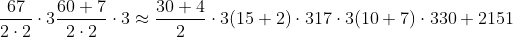67 USD is approximately 51 EUR. The exact answer is 50.25 EUR.

What you need to remember is thus:
1. The general operations: halve the number two times, then multiply by 3
2. How to halve and multiply by 3 using the "splitting" trick.
Let's we want to do the opposite now: convert euros into USD. 1/0.75 = 1.33, so 1 EUR = 1.33 USD.

Let's say we have 247 EUR. This is 247*1.33 USD, but how do we calculate it easily?

First, we omit the second decimal, i.e. 1.33 is approximately 1.3. So we only need to do 247 * 1.3. First, using the splitting trick, we see that: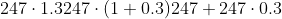So the hard part is a little easier now: we need to estimate 247*0.3. Noting that 0.3 = 3/10, and doing the division before multiplication as before, we get: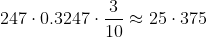So the answer is 247 + 75. A rough estimate is given by 250+75 = 250 + 50 + 25 = 325.

So 247 EUR is approximately 325 USD. The exact answer is 328.51 USD.

It seems then that the trick is to come up with ways to multiply and divide by decimal numbers, such as 0.75 and 0.3. This can always be done, since all conversion rates are rational numbers, meaning they can be written in the form Integer/Integer. By using a fairly simple numerator and denumerator, we can then perform the estimation fast. Multiplication is in general easy, division much harder. Here's a couple of tricks:

Divide by 2 (halving)
This is simply taking half the quantity. If you need to divide say 573 by 2, then use the splitting technique: 573 = 500 + 70 + 3. Halving each gives approximately 250 + 35 + 2 = 250 + 37 = 287.

Divide by 4 and 8 (halving many times)
Also very easy: dividing by 4 is the same as halving twice. Dividing by 8 is the same as halving three times.

Divide by 10
The easiest to do. 128 divided by 10 is 12.8. In general, XYZ divided by 10 will be XY.Z, where X,Y and Z are digits (0,1,2,3,...,9). We then simply round to nearest integer> 12.8 is closest to 13.

Divide by 5 ("divide by 10 and double")
This one's really easy too: x/5 = x/10*2, so all you need to do is divide by 10 (using the method above), and then double the result. Example: 128/5 can be calculated first dividing 128 by 10, giving 13. Doubling gives 26.

Divide by 3 ("take average of 2 and 5")
This one's a bit harder. A nice way to estimate x/3 that builds on the techniques already mentioned is to take the average of x/2 and x/5.
Example: 175 divided by 3 can be approximated by noting that:
175/5 = 18*2 = 36
175/2 = (100 + 75 + 5) / 2 = 50 + 37 + 3 = 50 + 40 = 90
Taking averages, we get (36+90)/2 = 126/2 = (100+20+6)/2 = 50 + 10 + 3 = 63.
In general, this technique gives (x/2 + x/5)/2 = 7x/20 = 0.35x, instead of 0.3333x.
(It may seem more intuitive to take average of x/2 and x/4, but this gives a worst estimate of 0.375x.)

Divide by 7 ("take average of 5 and 10")
Also a bit harder. When doing x/7, we can take the average of dividing by 5 and 10.
384/7 is somewhere between:
384/10 = 38
384/5 = 38*2 = (30+8)*2 = 60 + 16 = 76
Taking averages, we get (38+76)/2 = (100 + 14)/2 = 50 + 7 = 57
The exact answer is close to 55.
This techniques calculates (x/5 + x/10)/2 = 3x/20 = 0.15x instead of x/7 = 0.143, so it's a good estimate for relatively small sums of money.

Here's another example. 1 THB (Thai Baht) is 0.032 USD. How much is the saleswoman asking for in USD when she says 650 THB? The answer is 650*0.032 USD. To estimate this quantity, we manipulate the conversion rate 0.032 into rules of the form above. Note that 0.032 = 32/1000 which we approximate as 30/1000 = 3/100 (32/1000 is (30+2)/1000 = 3/100 + 2/1000, so we're skipping a term containing two parts in 1000).

Calculating 650 * 3/100 is easy: do the division first (dividing by 100 is dividing by 10 two times, since 100 = 10*10). We then get 6.5, which we round to 7. Multiplying by 3 gives 21. Here's the full estimate in one line: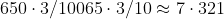Let's now convert from GBP (Brittish pound) to USD. 1 GBP = 1.56 USD. How much is Harrods asking you in USD when they say 489 GBP?

First we deal with the conversion rate. 1.56. This is close to 1.6, which we instead use. 1.6 = 16/10, and looking at prime factors we have: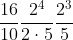This works: we can divide by 5 and then double 3 times. It's however rather tedious, as if we allow a little more rounding error, 1.56 is also close to 1.5, which gives: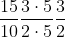A much simpler calculation: we half, then multiply by 3.
Thus, estimating 489 GBP is done as follows:
489/2 = (400 + 80 + 9)/2 = 200 + 40 + 5 = 245.
245 * 3 = (200 + 40 + 5)*3 = 600 + 120 + 15 = 720 + 15 = 735.

489 GBP is approximately 735 USD (although for such large amounts I would suggest you use a calculator!).

## Propositional Logic

In propositional logic (equivalently, boolean algebras), a common problem is to find a true/false assignment to all boolean variables such that all formulas are satisfied.

In mathematics, we would call this a solution, in logic, it's called a model:

2x + 3y = 16 has an integer solution {x=5, y = 2} (there are infinitely many integer solutions).
x and not y  has a model {x = true, y = false}

In general, the problem of finding models to propositional formulas is NP-complete.
It is by many believed to be exponential: in the worst case, the best known algorithms do no better than the truth table method of trying out all 2^N possibilties, where N is the number of boolean variables (in practice DPLL based algorithm like Chaff perform significantly better across almost all problems).

There are however a few subclasses of problems that are linear time solvable. Two such that have practical value are Horn and Dual Horn Clause logic, which we consider here.

If you don't know it yet, all propositional formulas can be written in Conjunctive Normal Form, which you'll need to know about before reading on.

## Horn Clause Logic (propositional)

Horn clauses are clauses that contain at most one positive literal.
I'll assume that the empty clause is not part of the database: if it is, there's obviously no model (this can be checked in linear time by simply traversing all clauses).

Let's start with the case where all clauses have no positive literals. Then all literals in all clauses are negative, so there is no conflict between any literals: all we need to do in order to satisfy all clauses is set all variables to false.

Now assume some (or all) clauses have a positive literal. If they also have a negative literal, we're back to our previous situation: by setting all literals to false, all clauses (include these new ones) are satisfied.

So the problem is when we have unit clause with one positive literal (and therefore, no negative literals). Clearly, we have no choice but to set these literals to true. This triggers a cascade of inferences by which other literals become unit. For example:

If we have the unit clause [X] and [ -X, Y ], we have no choice but to set X=1.
Then the second clause reduces to [ Y ], which in turn propagates Y=1.
And so on. This process is known as unit propagation.

After we've finished unit propagating, all necessary variable assignments have been performed. For the remaining variables, we have the same situation as before: they occur in non-unit clauses, so we can trivially handle them by assigning everything else to false. The algorithm is thus:

1. Unit Propagate (this may fail, e.g. if we have [X] and [-X], whereby there is no model).
2. Set remaining variables to false.

## Linear Time Unit Propagation

The obvious algorithm for unit propagation is to pick all unit clauses, put the literal in a queue (or stack, it doesn't matter), and then go through the queue of literals doing:

1. Eliminate all clauses containing the literal (this step can be omitted if we use two literal watch, see below).
2. Remove negated literal from all clauses.
3. Scan for new unit clauses, put literal in queue and add to model.
4. Repeat until queue is empty.

This is a loop nested inside another loop: for each literal (in the queue), we linearly scan all clauses to simplify them (step 1 and 2 above). Thus the complexity is O(N^2). The second step, of scanning through all literals, can be avoided by keeping a mapping from all literals (positive and negative literals are distinct) to clauses.

For example:
C1 = [X]
C2 = [ -X, Y ]
C3 = [ -X, -Y ]

Will give the table:
X : C1
Y : C2
-X: C2, C3
-Y: C3

In step 1 and 2 above, we can thus directly go to the clauses containing the literal (step 1) and its negation (step 2). Note that if this table is stored as a std::map, it will have lookup time log(N). If we use a hash map (with linear chaining, i.e. std::unordered_map), we will ideally get O(1) but worst case will be O(N). A way to get a truly O(1) lookup is to store the literals in a jump table so that we can immediately get a list of the clauses (std::vector<std::vector<clause*>>).

This presumes that we remove clauses/literals. If we use lazy datastructures, e.g. two literal watch, the obviously implementation of scanning for another available literal to watch yields a worst case of O(N) operations per literal deletion, so we end up having a O(N^2) worst case anyway. (That is, the obvious algorithm that had O(N^2) would actually be O(N^3) using two literal watch.) This is perhaps mostly academic, as collisions between assignments and watch literals are rare in large clauses. One could add another table to keep track of all available literals for each clause, making updating watched literals O(1). However, this benefit probably translate into worst performance when using the full DPLL algorithm on general problems, as backtracking would be more expensive: now we need to add/remove literals from this new table during every assignment/backtracking.

There is paper about linear time unit propagation here. It uses two literal watch and assumes that literals are updated in constant time.

## What about Dual Horn Logic?

In dual Horn logic, all clauses have at most one negative literal (instead of one positive, as in Horn logic).
So if we have non-unit literals, there must be at least one positive literal, and setting all variable assignments to true will thus be a model. If we have unit clauses, we unit propagate. The algorithm is:

1. Unit Propagate (may fail, see Horn clause example).
2. Assign True to all remaining variables.
So the only difference from Horn logic is that after unit propagation, we assign true to all remaining literals instead of false.

## Can DPLL solve Horn and Dual Horn Logic in Linear time?

Yes, but only if we use the selection strategy that (first) sets all variables to false for Horn logic, and true for dual Horn logic. This works since the DPLL is essentially:
1. Unit Propagate.
2. If propagation fails, backtrack. Go to step 3.
3. If there are no more literals to assign, return the model.
4. Select unassigned variable (according to selection strategy). Go to step 1.
If DPLL's backtrack fails, it will exit with a "no models available". It's easy to see that DPLL properly handled the Horn (Dual Horn) logic cases for these particular selection strategies, although with an overhead: after each assignment in step 4, DPLL tries to unit propagate, although no such propagation actually takes place. It then re-enters step 4 and assigns false (or true) to the next literal. This process is repeated until all remaining literals are set to false (true). In the efficient algorithms above: all remaining variables are directly set to false (true) with no unnecessary unit propagation between every assignment.

As mentioned before, there may be issues with true linear time solvability when using two literal watch with schemes that are efficient for backtracking (O(N) updating) vs schemes that are efficient for quickly updating watched literals (O(1) updating, but constant time penalty when propagating and backtracking).

The problem is that DPLL doesn't know that these assignments are safe in the Horn and Dual Horn cases (they are not "safe" in general, unit propagation is necessary, and this may trigger backtracking or solve the problem entirely).

So intuitively, the reason why Horn and Dual horn logic are linear time solvable whereas the general case is exponential is because of choice points and backtracking: in the general case, we need to make guesses, and if these guesses are wrong, we need to backtrack and try the other branch. So we are forking the possible paths repeatedly. With Horn and Dual horn logic, no guesses need to be made, and hence, no backtracking occurs.

## What about First-order Horn Clause Logic?

It is semi-decidable.

Proof: one can encode integers and basic arithmetic operations in Prolog. Thus general diophantine equations can be expressed, and a query can be made that succeeds if and only if it has a solution. If queries would be decidable, this would give a decision procedure for diophantine equations, contradicting Matiyasevich's theorem. So it is undecidable. That it is semi-decidable now follows from the Church-Turing theorem.

## Wednesday, April 17, 2013

You've almost certainly heard some of these (logical) paradoxes. They're fun brain puzzles, and people like to share them. Surprisingly, most people who share them do not know their solution, nor try to solve them. Here are some of the most well known paradoxes and their solutions.

Note that these are paradoxes of more logical nature (i.e. "mind puzzles"), and consequently, their solutions explore logic and language.

There are also paradoxes within scientific theories, with scientific solutions, which I might write about another time.

### Problem

Perhaps the most well known paradox. The version of the paradox that is the most clear is the following. Consider the sentence: "This sentence is false". We are wondering whether the sentence itself is true or false.

Assume the sentence is true.
Then clearly the sentence is false, a contradiction.

Now assume the sentence is false.
Then the sentence is true, also a contradiction.

It seems we cannot assign a binary truth value (true/false) to the sentence.

This paradox is sometimes given in the form: A person says that he is lying. Is he telling the truth or a lie?

### Solution

There are two things that are unusual about the sentence.

First, it makes a reference to itself, by using the word "this sentence". This is known as self reference, and the statement is said to be self-referential.

Second, the sentence assumes that it can express the truth/falsehood of other statements.

To see this, imagine that we were to express the sentence in a more formal language. It would look something like:

Sentence 1: not is_true(get_sentence(1))

In other words, we are assuming that we can get a sentence by it's label ("get_sentence(1)", which is the sentence itself, since we gave it label 1) and that we have a predicate "is_true" which returns true/false accurately (we need not assume anything about the computability of these functions, only that they are definable within the language).

If you haven't studied logic you might be inclined to think that the first point is a pretty serious problem whereas the second is trivial. You'd be wrong, however, because it's the other way around: assuming we can express "is false" is a much more problematic issue than using self references.

Here's why:
Self-referential statements are everywhere. I can easily get rid of the self reference in the liar paradox by doing the following:

Sentence 1: "Sentence 2 is true"
Sentence 2: "Sentence 1 is false"

If Sentence 1 is true, then both are true, which in turn means that Sentence 1 is false, a contradiction.
If Sentence 2 is false, then both are false, which in turn means that Sentence 1 is true, a contradiction.

You might feel like this point is moot, cause all I've done is replaced "this" with a reference to another sentence, which in turn references back to the first sentence. After all, decoupling the sentence does solve much since we can still express everything using get_sentence and is_true:

Sentence 1: is_true(get_sentence(2))
Sentence 2: not(is_true(get_sentence(1)))

That's a good point, except that the problem doesn't go away even if you forbid all kinds of references (to the language itself). In particular, almost any logical system can be re-encoded so that the sentences can be seen to implicitly contain self references. This is done using something called Gödel encodings, and it is roughly the equivalent of assigning every sentence in the English alphabet a unique number in a systematic way. get_sentence is easy to construct, even in languages where it was not intended to exist.

The same cannot be said for is_true: it does not in general exist. For example, arithmetic does not admit a truth predicate so there is no way of expressing "1+2=3 is true" in arithmetic. This result is known as Tarski's undefinability theorem.

You might wonder what all of this has to do with the English language, in which the paradox is presented. Well the point is that if we allow unrestricted use of natural languages (such as English), we can express pretty much anything. The problem is that many things we can express in English won't even be logical sentences, in the sense that they will not have truth values (such as the sentence under consideration). Unrestricted use of language also opens up the possibility for expressing nonsense.

What we can learn from the liar paradox is that we must clearly handicap our "language" if we wish to have a "no nonsense" language (e.g. predicate logic, modal logics, Horn clause logic). How do we handicap such languages to make sure the liar paradox doesn't appear? Gödel has shown us that there isn't much point in trying to remove self references; they occur very easily. Taski has shown us that a truth predicate "is true" is not usually available, so this is certainly more of a comfort.

In arithmetic, you cannot express sentences such as the liar paradox, not because it is self referential, but because it asserts the truth of arithmetical sentences. Put differenty, in arithmetic, is_true is not definable.

### Problem

The barber's paradox arises from the following assumption and question: A barber shaves everyone who doesn't shave themselves. Does he shave himself?

As we did for the liar paradox, we check to see which is true:

Assume the barber shaves himself.
Then he doesn't have himself, since he only shaves those that don't shave themselves.

Now assume the barber doesn't shave himself.
Then he shaves himself, a contradiction again.

### Solution

So this sentence is neither true nor false. As mentioned in the liar paradox, that is not so much of a problem in English, because we really shouldn't expect all sentences to even make sense. There is no problem in imagining a barber that shaves everyone who don't shave themselves, except that he shaves himself too. Or a barber that goes to another barber. The problem is within the confines of language, physically, there is no way to create the contradiction.

The lesson to learn from this was a hard one historically. Russell's paradox is a more formal (mathematical) version of the paradox, which has to do with set theory. Imagine that a set is a box. We can have an empty box, or put boxes inside empty boxes. So denote the empty box with { }. If we have something, X, inside a box, we'll write {X}. And if we have two things, like X and Y, we'll write {X,Y} and so on. Assume we're allowed to create boxes by (perhaps infinitely) repeating the following:

The empty box {} is a box.
{X,Y,...} is a box if X,Y,... are all boxes.

So we know that {} is a box, and { {} } is a box (the box that contains an empty box). Then we can put that box inside a box: { {{}}}. But these are not the only ways we can make boxes. We can put all three of these boxes into one box: { {}, {{}}, {{{}}} }. If you're wondering why this is useful to do in mathematics, it's because from this you can create all numbers. For example, define:

0 = {}
1 = {0} = { {} }
2 = {0,1} = { {}, {{}} }
3 = {0,1,2} = { {}, {{}}, {{},{{}}} }
...

These seems all good, until you define the following box (set):

B is the box containing all boxes that do not contain themselves. Does it contain itself?

What is meant by a box that contains itself? It quite literally means that you can find a copy of the box inside the box. If you think that's impossible, it's because you're thinking only about finite boxes. If we have infinite nesting, such as {{{{...}}}}, then if we "box it", i.e. wrap it around one more { }, we can see that the box has a "copy of itself". So let X = {{{....}}}. Then {X} is a box containing itself, but so is {X,X}, {X,{}}, {X,{},{{}}}, and so on. So there's not just one box that contains itself, but an infinite number.

Now back to the paradox: Does B contain itself?
Assume it does. Then B is inside B, i.e. B = {B,...}. But B is the box containing all boxes (say X) that do not contain themselves. Since B contains B, B cannot be X here. Therefore B does not contain itself.
Now assume B does not contain B. Then, since B contains all X that do not have X, and B is precisely such an X, B contains itself.

So we have: B in B if and only if B not in B, i.e. the set contains itself if and only if it does not contain itself. It's usually said to be a contradiction, but it is technically not: you can't prove "B in B" or "B not in B", only that "B in B iff B not in B". It's only a contradiction if you assume that the sentence must be true or false.

Regardless, it is an undesirable feature of a logical system to say the least.
So these simple rules to create boxes actually lead to the possibility of creating a sentence that is neither true nor false (or both). What set theorists learnt from this is that the creation of boxes (sets) must be restricted (see Von Neumann Universe for three intuitive rules that create sets without this paradox).

Technical note: Boxes are defined so that they can contain multiple copies, e.g. { {}, {} }, whereas (non-fuzzy) sets either contain or do not contain an element. Boxes are in fact multisets. None of this changes the argument however: from sets one can create multisets, and vice versa.

### Problem

Zeno's paradox has many forms, here's one that is easy to understand: A man is going to walk 100 meters. To reach there, he must first cross 50 meters. But to reach that, in turn, he needs to cross 25 meters. It's easy to see that when we keep halving the distances, we get increasingly smaller numbers (but never exactly zero). This suggests that the man most move "infinitely much" in order to reach the 100 meters (in fact, in order to reach anywhere at all!).

### Solution

The paradox itself makes some questionable assumptions about the nature of reality. More specifically, it assumes that we can divide distances into halves indefinitely. The existence of a smallest measurable length, the Planck length, certainly puts doubt on this.

But even if we accept the premiss, one could ask where the problem really is anyway.
Is it that we find it unreasonable that he can move an infinite number of times? We have already accepted that there's infinitely much space to move in, so perhaps we should also accept that he can move infinitely much (in finite time), especially since the distances are getting smaller and smaller. Mathematically, he must move: 100/2 + 100/4 + 100/8 + 100/16 + ... = 100 meters. So if we can complete the infinite series, he'll be just fine. From this point of view, Zeno's paradox really illuminates its own assumptions:

1. Space is continuous (we have infinitely many steps to move through).
2. Time is discrete (we are not allowed to pass through all infinite steps).

Modern science suggests both are discrete, in which case there's no problem: at each discrete time step, the person moves forward a finite number of discrete steps. And as shown above, if both are continuous, there isn't any problem either.

Turning the paradox against itself, we can observe that we can indeed move, so the combination of continuous space and discrete time seems incorrect as far as reality goes.

## Thursday, March 7, 2013

### Efficient Memoization using Partial Function Application

Memoization is any technique of storing previously computed values for fast retrieval, rather than recomputing the same value multiple times:

bool f(int); // defined elsewhere
f(2); // compute f(2)
f(2); // retrieve previously stored value for f(2) instead of recomputing it

This is useful when calling f is expensive.

In C++, the most obvious way to implement memoization is perhaps using a std::map:

(I'm assuming correctness is important here, hence, storing only hashes and return values won't do.)

bool f(int); // defined elsewhere
bool memo_f(std::map<int,bool>& memo_tbl, int i)
{
auto at = memo_tbl.find(i);
if (at == memo_tbl.end()) {
// Not previously computed, compute and store
at = memo_tbl.insert(std::make_pair(i,f(i))).first;
}
return at->second;
}

This works fine for any function from a domain X (int above) to another domain Y (bool above).
But what if we want to compute a function that takes multiple arguments?
Say we have:

bool f(int,int); // defined elsewhere

We could then use a std::map<std::pair<int,int>,bool>, where the pair represents the two arguments (more generally, we could use a std::tuple, or even a std::vector for variable size arguments).
But these approaches all have one problem that becomes apparent if we replace our int's with heavier objects:

bool f(const Matrix&, const Graph&);

bool memo_f(std::map<std::pair<Matrix,Graph>,bool>& memo_tbl, const Matrix& m, const Graph& g)
{
std::pair<Matrix,Graph> p(m,g); // <-- ouch! Copies the entire matrix and graph!
auto at = memo_tbl.find(p);
if (at == memo_tbl.end()) {
// Not previously computed, compute and store
at = memo_tbl.insert(std::make_pair(i,f(i))).first;
}
return at->second;
}

I'm assuming that all objects can be ordered. The argument works equally well using std::unordered_map with a hash function for all objects. How to order or hash objects is not the concern here, but rather, avoiding unnecessary copying of large objects.

Using points to solve the problem isn't a good idea:

bool f(const Matrix&, const Graph&);
typedef std::pair<const Matrix*,const Graph*> inputs;
// pair_ptr_cmp defined elsewhere, dereferences the pointers inside the pair and compares them

bool memo_f(std::map<inputs,bool,pair_ptr_cmp>& memo_tbl, const Matrix& m, const Graph& g)
{
inputs p(&m,&g);
auto at = memo_tbl.find(p);
if (at == memo_tbl.end()) {
// Not previously computed, compute and store
bool ans = f(*m,*g);
// Note safe to store soft pointers to external objects, so we need to copy
p = inputs(new Matrix(*m),new Graph(*g));
at = memo_tbl.insert(std::make_pair(p,ans)).first;
    }
return at->second;
}


This works, except we need to define the awkward pair_ptr_cmp, and, the entire block starting right after our declaration of memo_tbl (not shown above) until it won't be used anymore will have to be wrapped in a try - catch (...) block:

std::map<inputs,bool,pair_ptr_cmp> memo_tbl;
try {
// Want to call f with Matrix m and graph g
bool b = memo_f(memo_tbl, m,g);
// ...
} catch (...) {
for (auto& p : memo_tbl) {
delete p.first;
delete p.second;
}
throw;
}


Needless to say, this is hard to read, error-prone, and just bad C++.

A good solution I've found is to use the concept of partial function application. The idea is simple: we may curry a 2 dimensional function (X,Y) -> f(X,Y) as X -> (Y -> f(X,Y)). That is, instead of taking two arguments from domain (of type) X and Y, it takes one argument from X and returns a function. This new function, takes the second argument, Y, and returns the desired f(X,Y).
Some code will make the point clear, and also demonstrate its elegance:

typedef std::string arg1_type;
typedef std::vector<int> arg2_type;
typedef bool ret_type;

// Function to call
bool function(const arg1_type& a1, const arg2_type& a2);

// Memoization of function
typedef std::map<arg1_type,std::map<arg2_type,ret_type>> memo_tbl;
ret_type memo_call(memo_tbl& f, const arg1_type& a1, const arg2_type& a2);

ret_type memo_call(memo_tbl& f, const arg1_type& a1, const arg2_type& a2)
{
ret_type ans;
auto at = f.find(a1);
if (at != f.end()) {
auto at2 = at->second.find(a2);
if (at2 != at->second.end()) {
ans = at2->second;
} else {
// map for a1 exists but value of a2 not stored
ans = function(a1,a2);
at->second.insert(std::make_pair(a2,ans));
}
} else {
// map for a2 (and a2) does not exist
ans = function(a1,a2);
std::map<arg2_type,ret_type> m;
m.insert(std::make_pair(a2,ans));
f.insert(std::make_pair(a1,std::move(m)));
}
return ans;
}


We can now test it:

static int counter = 0; // function keeps track of number of calls
bool function(const arg1_type& a1, const arg2_type& a2)
{
++counter;
return a1.size() == a2.size();
}

int main()
{
memo_tbl memo_tbl;
std::cerr << memo_call(memo_tbl,arg1_type("Hello World!"),arg2_type(10)) << "\n";
std::cerr << memo_call(memo_tbl,arg1_type("Hello World!"),arg2_type(12)) << "\n";
std::cerr << memo_call(memo_tbl,arg1_type("Hello World!"),arg2_type(10)) << "\n";
std::cerr << memo_call(memo_tbl,arg1_type("Bye World!"),arg2_type(10)) << "\n";

std::cerr << "Counter: " << counter << "\n";
}

Output is:
0
1
0
1
Counter: 3

The method generalizes to n-arity functions in the obvious way: just nest std::maps inside each other.